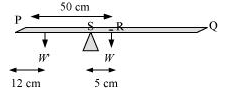## Pages

### System of particles and Rotational motion NCERT Solutions Class 11 Physics - Solved Exercise Question 7.17

Question 7.17:
A metre stick is balanced on a knife edge at its centre. When two coins, each of mass 5 g are put one on top of the other at the 12.0 cm mark, the stick is found to be balanced at 45.0 cm. What is the mass of the metre stick?
Solution:
Let W and W′ be the respective weights of the metre stick and the coin.The mass of the metre stick is concentrated at its mid-point, i.e., at the 50 cm mark.
Mass of the meter stick = m
Mass of each coin, m = 5 g
When the coins are placed 12 cm away from the end P, the centre of mass gets shifted by 5 cm from point R toward the end P. The centre of mass is located at a distance of 45 cm from point P.
The net torque will be conserved for rotational equilibrium about point R.
10 X g(45 - 12) - m'g(50 - 45) = 0
∴ m' = 66 g
Hence, the mass of the metre stick is 66 g.